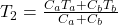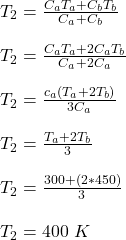The heat capacity of object B is twice that of object A. Initially A is at 300 K and B at 450 K. They are placed in thermal contact and the

Question

The heat capacity of object B is twice that of object A. Initially A is at 300 K and B at 450 K. They are placed in thermal contact and the combination is thermally insulated. The final temperature of both objects is

in progress 0
5 months 2021-08-10T01:48:53+00:00 1 Answers 208 views 0

The final temperature of both objects is 400 K

Explanation:

The quantity of heat transferred per unit mass is given by;

Q = cΔT

where;

c is the specific heat capacity

ΔT is the change in temperature

The heat transferred by the  object A per unit mass is given by;

Q(A) = caΔT

where;

ca is the specific heat capacity of object A

The heat transferred by the  object B per unit mass is given by;

Q(B) = cbΔT

where;

cb is the specific heat capacity of object B

The heat lost by object B is equal to heat gained by object A

Q(A) = -Q(B)

But heat capacity of object B is twice that of object A

The final temperature of the two objects is given byBut heat capacity of object B is twice that of object ATherefore, the final temperature of both objects is 400 K.